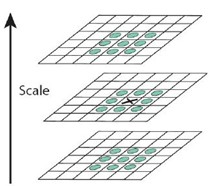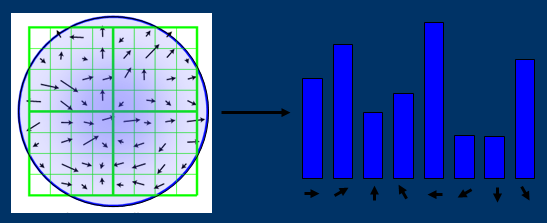LOG,HOG,DOG微分算子在近圆的斑点检测方面效果很好

### HOG特征

总结：Dalal提出的Hog特征提取的过程：把样本图像分割为若干个像素的单元（cell），把梯度方向平均划分为9个区间（bin），在每个单元里面对所有像素的梯度方向在各个方向区间进行直方图统计，得到一个9维的特征向量，每相邻的4个单元构成一个块（block），把一个块内的特征向量联起来得到36维的特征向量，用块对样本图像进行扫描，扫描步长为一个单元。最后将所有块的特征串联起来，就得到了人体的特征。

### LOG特征

图像与某一个二维函数进行卷积运算实际就是求取图像与这一函数的相似性。同理，图像与高斯拉普拉斯函数的卷积实际就是求取图像与高斯拉普拉斯函数的相似性。当图像中的斑点尺寸与高斯拉普拉斯函数的形状趋近一致时，图像的拉普拉斯响应达到最大。

Laplace可以用来检测图像中的局部极值点，但是对噪声敏感，所以在我们对图像进行Laplace卷积之前，我们用一个高斯低通滤波对图像进行卷积，目标是去除图像中的噪声点

先对图像f(x,y)用方差为σ的高斯核进行高斯滤波，去除图像中的噪点。

L(x,y;σ)=f(x,y)∗G(x,y;σ)
G(x,y;σ)高斯核

∇^2=(∂^2L/∂^x2)+(∂^2L/∂y^2)

∇^2[G(x,y)∗f(x,y)]=∇^2[G(x,y)]∗f(x,y)

### Haar特征

Haar特征分为三类：边缘特征、线性特征、中心特征和对角线特征，组合成特征模板。特征模板内有白色和黑色两种矩形，并定义该模板的特征值为<h3>白色矩形像素和减去黑色矩形像素和</h3>

### Haar-like特征

https://blog.csdn.net/zouxy09/article/details/7929570

积分图就是只遍历一次图像就可以求出图像中所有区域像素和的快速算法，大大的提高了图像特征值计算的效率。

积分图主要的思想是将图像从起点开始到各个点所形成的矩形区域像素之和作为一个数组的元素保存在内存中，当要计算某个区域的像素和时可以直接索引数组的元素，不用重新计算这个区域的像素和，从而加快了计算（这有个相应的称呼，叫做动态规划算法）。积分图能够在多种尺度下，使用相同的时间（常数时间）来计算不同的特征，因此大大提高了检测速度。

我们来看看它是怎么做到的。

积分图是一种能够描述全局信息的矩阵表示方法。积分图的构造方式是位置（i,j）处的值ii(i,j)是原图像(i,j)左上角方向所有像素的和：

i¯(x,y)=(i(x,y)−μ)/cσ

σ2=(1/N)∑x2−μ2   2是平方

### SIFT特征

SIFT的全称是Scale Invariant Feature Transform，尺度不变特征变换(物体怎么转，人都能识别)。SIFT特征对旋转、尺度缩放、亮度变化等保持不变性，是一种非常稳定的局部特征。

1. 尺度空间的极值检测 搜索所有尺度空间上的图像，通过高斯微分函数来识别潜在的对尺度和选择不变的兴趣点。
$L(x,y,σ)=G(x,y,σ)∗I(x,y)$

$D(x,y,σ)=(G(x,y,kσ)−G(x,y,σ))∗I(x,y)=L(x,y,kσ)−L(x,y,σ)$

σ 是尺度坐标。σ大小决定图像的平滑程度，大尺度对应图像的概貌特征，小尺度对应图像的细节特征。大的σ值对应粗糙尺度(低分辨率)，反之，对应精细尺度(高分辨率)。1. 特征点定位 在每个候选的位置上，通过一个拟合精细模型来确定位置尺度，关键点的选取依据他们的稳定程度。
拟和三维二次函数以精确确定关键点的位置和尺度，同时去除低对比度的关键点和不稳定的边缘响应点(因为DoG算子会产生较强的边缘响应)，以增强匹配稳定性、提高抗噪声能力

1. 特征方向赋值 基于图像局部的梯度方向，分配给每个关键点位置一个或多个方向，后续的所有操作都是对于关键点的方向、尺度和位置进行变换，从而提供这些特征的不变性。
每个特征点计算一个方向，依照这个方向做进一步的计算， *利用关键点邻域像素的梯度方向分布特性为每个关键点指定方向参数，使算子具备旋转不变性。

$m(x,y)=(L(x+1,y)−L(x−1,y))2+(L(x,y+1)−L(x,y−1))2$

$√θ(x,y)=atan2(L(x,y+1)−L(x,y−1)L(x+1,y)−L(x−1,y)$1. 特征点描述 在每个特征点周围的邻域内，在选定的尺度上测量图像的局部梯度，这些梯度被变换成一种表示，这种表示允许比较大的局部形状的变形和光照变换。

### 尺度空间

1. 对原始图像进行平滑
2. 对处理后的图像进行降采样（通常是水平、垂直方向的1/2）降采样后得到一系列不断尺寸缩小的图像。显然，一个传统的金字塔中，每一层的图像是其上一层图像长、高的各一半。多分辨率的图像金字塔虽然生成简单，但其本质是降采样，图像的局部特征则难以保持，也就是无法保持特征的尺度不变性。

图像的模糊程度来模拟人在距离物体由远到近时物体在视网膜上成像过程，距离物体越近其尺寸越大图像也越模糊，这就是高斯尺度空间，使用不同的参数模糊图像（分辨率不变）

L(x,y,σ)=G(x,y,σ)∗I(x,y)

G(x,y,σ)=（1/2Πσ^2）e^((x^2+y^2)/(2σ^2))

### DoG特征

DOG可以看作为LOG的一个近似，但是它比LOG的效率更高。

D(x,y,σ)=[G(x,y,kσ)−G(x,y,σ)]∗I(x,y)=L(x,y,kσ)−L(x,y,σ)

L(x,y,σ) 是图像的高斯尺度空间

### Harris角点特征提取

<font color=red>Harris角点检测是一种基于图像灰度的一阶导数矩阵检测方法。检测器的主要思想是局部自相似性/自相关性，即在某个局部窗口内图像块与在各个方向微小移动后的窗口内图像块的相似性。</font>

1、角点可以是两个边缘的角点；

1. 角点是邻域内具有两个主方向的特征点；
人眼对角点的识别通常是在一个局部的小区域或小窗口完成的。如果在各个方向上移动这个特征的小窗口，窗口内区域的灰度发生了较大的变化，那么就认为在窗口内遇到了角点。如果这个特定的窗口在图像各个方向上移动时，窗口内图像的灰度没有发生变化，那么窗口内就不存在角点；如果窗口在某一个方向移动时，窗口内图像的灰度发生了较大的变化，而在另一些方向上没有发生变化，那么，窗口内的图像可能就是一条直线的线段。

$x^{y^z}=(1+{\rm e}^x)^{-2xy^w}$

$sqrt()$

1. Harris角点检测算子对亮度和对比度的变化不敏感
2. Harris角点检测算子具有旋转不变性
3. Harris角点检测算子不具有尺度不变性